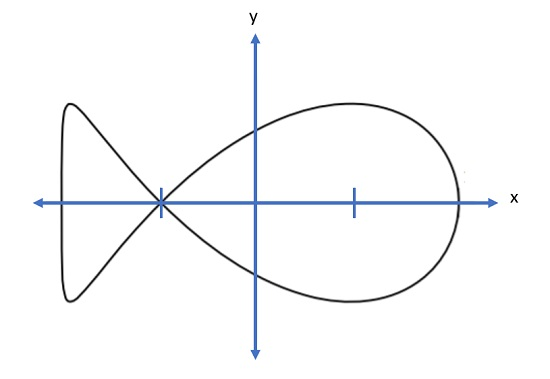# Fish Curve: Definition, Examples

## What is a Fish Curve?

The fish curve, more formally the ellipse negative pedal curve, is a quartic curve that gets its name because it looks like the shape of a fish.In the above image, one double point separates the “head” and “tail”. However, fish curves can take on many different shapes as it is created from stretched, segmented ellipses. For example, this next image of a fish curve has three singularities:

More formally, a fish curve is the negative pedal of an ellipse with the pedal point at the focus for eccentricity e2 = ½ ; “Eccentricity” tells you how much a curve deviates from a circle, which has an eccentricity of 0.An ellipse [2, √2] and its negative pedal curves with foci at (±√2, 0).

## What is the Pedal of a Curve?

The fish curve, aka the ellipse negative pedal curve is, as the name suggests, a curve with a negative pedal. A pedal is a specific type of locus; a pedal curve is drawn from a point to a certain geometric figure like a triangle . More precisely, the pedal curve of an arbitrary curve with respect to a fixed point (called the pedal point), is the locus of the point of intersection from O to one of the curve’s tangent lines .## Equations for the Fish Curve

The parametric equations for the fish curve depend on the shape of the ellipse on which the curve is based. For example, given an ellipse with the parametric equationsthe resulting fish curve will have the parametric equations## References

Pedal curve image: Woshiqiqiye, , CC BY-SA 3.0 via Wikimedia Commons.
 Tan, S. Handbook of Famous Plane Curves Using Mathematica.
 Jeon, K. What is a Pedal Curve? Retrieved December 27, 2021 from: http://jwilson.coe.uga.edu/emt669/Student.Folders/Jeon.Kyungsoon/document1/Essay1.html
 Pedal Curves. Retrieved December 27, 2021 from: https://mse.redwoods.edu/darnold/math50c/CalcProj/Fall98/NateB/pedal.htm

CITE THIS AS:
Stephanie Glen. "Fish Curve: Definition, Examples" From StatisticsHowTo.com: Elementary Statistics for the rest of us! https://www.statisticshowto.com/fish-curve-definition-examples/